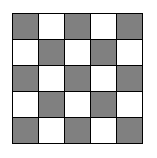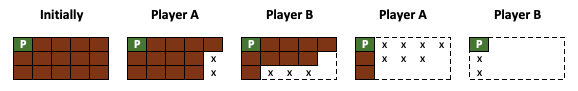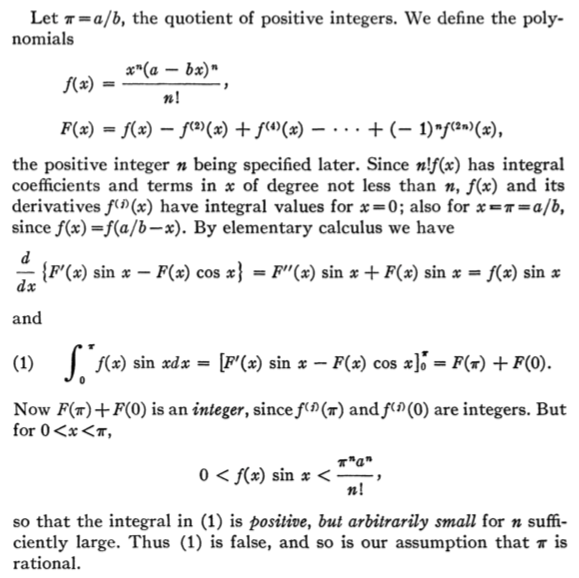As needed in the problems below, you may assume that for any prime $p$,

if $p \mid nm$, then $p \mid n$ or $p \mid m$.

1. We argue by cases:

Consider the possible remainders produced when $p$ is divided by 3. The only possibilities are 0, 1, and 2. Consider each case separately: \begin{align*} p \textrm{ has remainder } 0 \textrm{ when divided by 3 }&\Rightarrow 3 \mid p\\ & \\p \textrm{ has remainder } 1 \textrm{ when divided by 3 }&\Rightarrow p = 3k+1\\ &\Rightarrow p+2 = 3k+3\\ &\Rightarrow p+2 = 3(k+1)\\ &\Rightarrow 3 \mid p+2\\ & \\p \textrm{ has remainder } 2 \textrm{ when divided by 3 }&\Rightarrow p = 3k+2\\ &\Rightarrow p+4 = 3k+6\\ &\Rightarrow p+4 = 3(k+2)\\ &\Rightarrow 3 \mid p+4\end{align*} Thus (with the exception of 3, 5, and 7) three consecutive odd numbers are never all prime. One of them must always be divisible by 3.

QED.

2. Let's try to argue indirectly...

Assume that $a+b$ is a rational number. Then we can find integers $m$ and $n$ where $$a+b=\frac{m}{n}$$ Since $a$ is also rational, we can find integers $s$ and $t$ where $$a = \frac{s}{t}$$ Consider what happens when we solve the first equation for $b$: \begin{align*}b &= \frac{m}{n} - a\\\\ &= \frac{m}{n} - \frac{s}{t}\\\\ &= \frac{mt}{nt} - \frac{ns}{nt}\\\\ &= \frac{mt-ns}{nt} \end{align*} Notice that both $mt-ns$ and $nt$ are integers. We have just written $b$ as the quotient of two integers. But then $b$ must be rational -- which contradicts the given fact that $b$ is irrational.

As such, our assumption must be rejected, and the opposite must be true: $a+b$ is an irrational number.

QED.

We can tighten this argument up substantially if we instead appeal to the closure of the rational numbers under subtraction:

Again, assume that $a+b$ is rational. That is to say:

$$a+b = r \quad \textrm{ for some } r \in \mathbb{Q}$$

But then $b = r - a$, so $b$ is a difference of rational numbers and must then be rational itself as $\mathbb{Q}$ is closed under subtraction. We have just contradicted the given irrationality of $b$.

As such, our assumption must be rejected, and the opposite must be true: $a+b$ is an irrational number.

QED.

3. Let's argue indirectly:

Assume that $\sqrt{2}$ is rational. Then we can find integers $m$ and $n$ where $$\sqrt{2} = \frac{m}{n} \quad \textrm{(which is in lowest terms)}$$ Cubing both sides, we find \begin{align*}2 = \frac{m^3}{n^3} &\Rightarrow 2n^3 = m^3\\ &\Rightarrow 2 \mid m^3\\ &\Rightarrow 2 \mid m\\ &\Rightarrow m = 2k_1 \quad \textrm{for some integer } k_1\end{align*} Now, substituting our new expression for $m$ into a previous equation, we find \begin{align*} 2n^3 = m^3 &\Rightarrow 2n^3 = (2k_1)^3\\ &\Rightarrow 2n^3 = 8{k_1}^3\\ &\Rightarrow n^3 = 2 \cdot 2{k_1}^3\\ &\Rightarrow 2 \mid n^3\\ &\Rightarrow 2 \mid n\end{align*} But this contradicts the assumption that we could find such a fraction $m/n$ in lowest terms (2 divides both $m$ and $n$).

Hence, our assumption must be rejected, and the opposite must be true: $\sqrt{2}$ is irrational.

QED.

4. We must first show that $\sqrt{2}$ is irrational.

To do this, argue indirectly and assume $\sqrt{2}$ is rational.

But then, we can find integers $m$ and $n$ where $$\sqrt{2} = \frac{m}{n} \quad \textrm{(which is in lowest terms)}$$ Squaring both sides, we find \begin{align*}2 = \frac{m^2}{n^2} &\Rightarrow 2n^2 = m^2\\ &\Rightarrow 2 \mid m^2\\ &\Rightarrow 2 \mid m\\ &\Rightarrow m = 2k_1 \quad \textrm{for some integer } k_1\end{align*} Now, substituting our new expression for $m$ into a previous equation, we find \begin{align*} 2n^2 = m^2 &\Rightarrow 2n^2 = (2k_1)^2\\ &\Rightarrow 2n^2 = 4{k_1}^2\\ &\Rightarrow n^2 = 2{k_1}^2\\ &\Rightarrow 2 \mid n^2\\ &\Rightarrow 2 \mid n\end{align*} But this contradicts the assumption that we could find such a fraction $m/n$ in lowest terms (2 divides both $m$ and $n$).

Hence, our assumption must be rejected, and the opposite must be true: $\sqrt{2}$ is irrational.

Now, we proceed to the main argument. Again, we argue indirectly...

Assume that $\sqrt{2}+\sqrt{5}$ is rational.

But then, we can find new integers $m$ and $n$ where

$$\sqrt{2} +\sqrt{5} = \frac{m}{n} \quad \textrm{(which is in lowest terms)}$$

Suppose we eliminate one of the radicals in the above equation, by squaring both sides... \begin{align*}n\sqrt{2} + n\sqrt{5} &= m\\ n\sqrt{5} &= m - n\sqrt{2}\\ 5n^2 &= (m - n\sqrt{2})^2\\ 5n^2 &= m^2 - 2mn\sqrt{2} + 2n^2\\ \end{align*} Now, "solving for $\sqrt{2}$", we find:\begin{align*}5n^2 + 2mn\sqrt{2} &= m^2 + 2n^2\\ 2mn\sqrt{2} &= m^2 - 3n^2\\\\ \sqrt{2} &= \frac{m^2-3n^2}{2mn} \end{align*} But noticing that both the numerator and denominator of the above fraction must be integers, we have just shown $\sqrt{2}$ is rational.

We know that this can't possibly be the case, given our earlier result. As such, our assumption that $\sqrt{2}+\sqrt{5}$ is rational must be rejected, and the opposite must be true: $\sqrt{2}+\sqrt{5}$ is irrational.

QED.

5. Prove that $\sqrt{2} + \sqrt{3} + \sqrt{5}$ is irrational.

Let's argue indirectly...

Assume that $\sqrt{2} + \sqrt{3} + \sqrt{5}$ is rational.

As such, we can find a rational number $r>0$ where $$\sqrt{2} + \sqrt{3} + \sqrt{5} = r$$ Suppose, following a natural instinct, we try to reduce the number of radicals in this equation. We can start by pulling the $\sqrt{5}$ to one side and then squaring both sides...

\begin{align*}\sqrt{2} + \sqrt{3} &= r - \sqrt{5}\\ (\sqrt{2} + \sqrt{3})^2 &= (r - \sqrt{5})^2\\ 2 + 2\sqrt{6} + 3 &= r^2 - 2r\sqrt{5} +5\\ 2\sqrt{6} &= r^2 - 2r\sqrt{5}\\ (2\sqrt{6})^2 &= (r^2 - 2r\sqrt{5})^2\\ 24 &= r^4 - 4r^3\sqrt{5} + 20r^2\\ 4r^2\sqrt{5} &= r^4 +20r^2 - 24\\ \sqrt{5} &= \frac{r^4 +20r^2 - 24}{4r^2}\end{align*}

Recall, non-zero rational numbers are closed with respect to addition, subtraction, multiplication, and division -- so $\dfrac{r^4 +20r^2 - 24}{4r^2}$ must be a rational number. But then, $\sqrt{5}$ must be rational. We know this can't be! We have our desired contradiction. As such, we must reject our assumption.

Instead, its opposite must be true: $\sqrt{2} + \sqrt{3} + \sqrt{5}$ is irrational.

QED.

6. Prove that $\sqrt{2} \cdot \sqrt{3}$ is irrational.

7. Prove that $\displaystyle{\frac{\sqrt{2}}{\sqrt{3}}}$ is irrational.

8. Let's argue indirectly...

Assume that all are acquaintances with a different number of people.

Notice, there are $n$ people and $n$ possibilities for the numbers of acquaintances each can have: $0,1,2,\ldots,n-1$.

If each person is acquainted with a different number of people, then each of these numbers must get used.

As such, there must be a person acquainted with everyone else ($n-1$ people), as well as a person with no acquaintances (0 people).

However, the person acquainted with everyone else can't be acquainted with the person who has no acquaintances, so unless $n-1=0$, which makes these two values associated with the same person (which happens when $n=1$) we have our contradiction.

As such, when $n \ge 2$, we must reject our assumption.

Instead, its opposite must be true: There must be two people with the same number of acquaintances.

QED.

9. Prove that $\displaystyle{e = 1 + \frac{1}{1!} + \frac{1}{2!} + \frac{1}{3!} + \frac{1}{4!} + \cdots}$ is irrational.
(Yes, this is the same $e$ used as a base in the natural logarithm function.)

Let's argue indirectly...

Assume that $e$ is rational. Then there exists integers $p$ and $q$ such that $\displaystyle{e = \frac{p}{q}}$.

Let $k$ be defined by

$$k = q! \left(e - 1 - \frac{1}{1!} - \frac{2}{2!} - \cdots - \frac{1}{q!} \right)$$

Clearly, $k$ is an integer as each denominator present divides $b!$ evenly. Further, $k$ is a positive, as $b!$ is a positive and the terms subtracted from $e$ sum to something less than $e$. Thus, $k$ is a positive integer.

But simplifying $k$, we have \begin{align*} k &= q! \left(e - 1 - \frac{1}{1!} - \frac{2}{2!} - \cdots - \frac{1}{q!} \right)\\ &= q! \left( \left( 1 + \frac{1}{1!} + \frac{1}{2!} + \cdots + \frac{1}{q!} + \frac{1}{(q+1)!} + \cdots \right) - 1 - \frac{1}{1!} - \frac{2}{2!} - \cdots - \frac{1}{q!} \right)\\ &= q! \left(\frac{1}{(q+1)!} + \frac{1}{(q+2)!} + \frac{1}{(q+3!)} + \cdots\right)\\ &= \frac{1}{(q+1)} + \frac{1}{(q+1)(q+2)} + \frac{1}{(q+1)(q+2)(q+3)} + \frac{1}{(q+1)(q+2)(q+3)(q+4)} + \cdots\\ &< \frac{1}{(q+1)} + \frac{1}{(q+1)^2} + \frac{1}{(q+1)^3} + \frac{1}{(q+1)^4} + \cdots\\ &= \frac{1}{q} \end{align*}

The last expression above is obtained by noting that the sum above it represents a geometric series with common ratio $r = 1/(q+1)$ whose sum is given by

$$\frac{\frac{1}{(q+1)}}{1 - \frac{1}{(q+1)}} = \frac{\frac{1}{(q+1)}}{\left(1 - \frac{1}{q+1}\right)} \cdot \frac{(q+1)}{(q+1)} = \frac{1}{(q+1)-1} = \frac{1}{q}$$ As such, we have shown that $k$ is a positive integer less than $1/q$ where $q$ is some integer, which is in turn less than one. This, of course is impossible -- we have the desired contradiction. Thus, $e$ must be irrational.

10. Let's try to argue by contradiction...

Assume that there does not exist a triangle with sides $a,b,c$.

Recalling that three segments can only form a triangle if the sum of the lengths of any two of them is larger than the length of the third, it must be the case that the sum of $a$ and $b$ must be less than or equal to $c$. Consequently, \begin{align*} a+b & \le c\\ (a+b)^2 & \le c^2\\ a^2 + 2ab + b^2 & \le c^2 \end{align*} Now, note that since $a$ and $b$ are positive, $2ab$ must also be positive. Thus, we can subtract $2ab$ from just the left side and still preserve the direction of the inequality, yielding $$a^2 + b^2 \lt c^2$$ Hence, there does not exist a triangle with sides $a^2,b^2,c^2$. But this contradicts the given fact that there does exist a triangle with these side lengths. Consequently, our assumption must be rejected, and the opposite must be true:

There exists a triangle with sides $a,b,c$.

QED.

Note, with some slight modification, we can also argue the result directly...

If there is a triangle with sides $a^2$, $b^2$, and $c^2$, it must be the case that

$$a^2 + b^2 \gt c^2$$

In considering a triangle of sides $a$, $b$, and $c$, we first note that $a$ and $b$ must be positive as they represent lengths. Consequently, $2ab > 0$ and we can add this value to the left side above, while preserving the inequality. Hence,

\begin{align*} a^2 + 2ab + b^2 &\gt c^2\\ (a+b)^2 &\gt c^2\\ a+b &\gt c \end{align*}

In a similar way, we can argue that it must be the case that $a^2 + c^2 \gt b^2$, which leads to $a+c \gt b$; and $b^2 + c^2 \gt a^2$, which leads to $b+c>a$. Since the sum of any two of the values $a$, $b$, and $c$ sum to something greater than the third, there does indeed exist a triangle with these side lengths.

QED.

11. Without loss of generality, suppose the board is colored in the following way:Let's argue indirectly...

Assume that, after the bugs move, there is no more than one bug in each square.

In particular, this means that there can be no more than 12 bugs on the white squares.

Notice that if the bugs moved to adjacent squares (by a side), then bugs that were on black squares are now on white squares and vice-versa. But all 13 black bugs moved to white squares, so there have to be more than 12 bugs on the white squares. We have a clear contradiction.

Consequently, our assumption must be rejected, and the opposite must be true: there is more than one bug in one of the squares.

QED.

12. Let's argue indirectly...

Assume there is no person who knows an even number of people.

Thus, all 7 people have an odd number of acquaintances.

If, for each person, we count the number of acquaintances, and then look at the total count, we see it is the sum of 7 odd numbers, which itself must be odd.

Now consider finding this total a different way: Notice that two people being acquaintances contributes 2 to the total we just found. (i.e., If person A knows person B, then person B also knows person A.) But then, being the sum of a bunch of 2's, the total in question must be even.

The total can't be both odd and even, so we have our desired contradiction.

Consequently, our assumption must be rejected, and its opposite must be true: there is a person who knows an even number of people.

QED.

13. With the exception of 2, we first note all primes must be of the form $4k+1$ or $4k+3$ where $k$ is an integer, as $2 \mid 4k + 0$ and $2 \mid 4k+2$.

Now, let's consider what happens when we multiply primes (or any numbers for that matter) of these forms together.

For example, the following shows that multiplying two numbers of the form $4k+1$ together yields another number of the same form.

$$\begin{array}{rcl} (4k_1 + 1)(4k_2 + 1) &=& 16k_1 k_2 + 4k_1 + 4k_2 + 1 \\ &=& 4(4k_1 k_2 + k_1 + k_2) + 1 \\ &=& 4k_3 + 1 \quad \textrm{ for some integer } k_3 \end{array}$$

When multiplying two numbers of the form $4k+3$, we again get a number of the form $4k+1$:

$$\begin{array}{rcl} (4k_1 + 3)(4k_2 + 3) &=& 16k_1 k_2 + 12k_1 + 12k_2 + 9 \\ &=& 4(4k_1 k_2 + 3k_1 + 3k_2 + 2) + 1 \\ &=& 4k_3 + 1 \quad \textrm{ for some integer } k_3 \end{array}$$

Multiplying a number of the form $4k+1$ by another of the form $4k+3$, however, results in a number of the form $4k+3$

$$\begin{array}{rcl} (4k_1 + 1)(4k_2 + 3) &=& 16k_1 k_2 + 12k_1 + 4k_2 + 3 \\ &=& 4(4k_1 k_2 + 3k_1 + k_2) + 3 \\ &=& 4k_3 + 3 \quad \textrm{ for some integer } k_3 \end{array}$$

Turning our attention back to the claim to be proven, let us now argue indirectly...

Assume that there are a finite number of primes of the form $4k+3$, namely:

$$p_1, p_2, p_3, \ldots , p_n$$

If $n$ is even, consider the value

$$q = p_1 p_2 p_3 \cdots p_n + 2$$

If $n$ is odd, consider the following instead

$$q = p_1^2 p_2 p_3 \cdots p_n + 2$$

In either case, we have an even number of primes of the form $4k+3$ multiplied together, producing a value of the form $4k+1$. With the additional "$+2$", it must be the case that $q$ is a number of the form $4k+3$.

Consequently, $q$ must have a prime divisor of the form $4k+3$. Consider the alternative, if all of its prime divisors were of the form $4k+1$, then it too would be of that form.

However, this contradicts the fact that $q$ has no prime divisors of this form, as primes of this form are precisely $p_1, p_2, p_3, \ldots, p_n$ and each of these leaves a remainder of $2$ when used to divide $q$.

Since our assumption led to a contradiction, we must reject it.

Hence, there are an infinite number of primes of the form $4k+3$, where $k$ is an integer.

14. Let's argue indirectly...

Assume that the king made no diagonal moves.

Then, on each move the king must have changed the color of its square.

Now consider the list of all squares, in order, according to when they were visited by the king. This list starts with a white square and ends with a white square, with the colors of the squares alternating in between. Hence, there must be an odd number of squares.

But the king visited all 64 squares exactly once. This is our contradiction.

Hence, our assumption must be rejected, and its opposite must be true: the king must have made at least one diagonal move.

15. 🔎 Prove that, given five consecutive positive integers, it is always possible to find one which is relatively prime to all the rest.

16. 🔎 Chomp is a game played by two players (player $A$ who goes first, and player $B$ who goes second) starting with a rectangle of delicious chocolate. The upper left square of the chocolate, though still delicious, is poisoned and the two players wish to avoid eating it. The game proceeds in turns. On a player's turn, he or she must choose one square of the chocolate and eats that square as well as all the squares below and to the right of it (including any squares directly below or to the right). The player who eats the poisoned square loses.

Below shows a sequence of moves in a typical game, starting with a $3 \times 5$ bar:In the last move, player $A$ must eat the last (poisoned) block and so loses.

One obviously wants to develop a strategy for avoiding being poisoned. As it turns out (which we will show with an induction argument later), one of the two players must have a winning strategy. That is to say -- one of the players, if they play perfectly, can win every time no matter what choices the other player makes.

Certainly this is true for a $1 \times 1$ board, where the winning strategy for player $B$ is simply to watch player $A$ poison himself.

Assuming one player must have a winning strategy, prove that for all boards larger than a $1 \times 1$ board, player $A$ has the winning strategy.

17. Prove that $\pi$ is irrational. (requires some knowledge of Calculus II)

Consider the following proof by Ivan Niven: